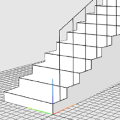# The Möbius Ecosystem

A web-based ecosystem for parametric modelling.

Möbius Grader is an open-source web-app for automated grading of parametric modelling assignments using Möbius Modeller.

The Möbius Grader is used for grading assignments for the Edx Spatial Computational Thinking Professional Certificate Programme.

Möbius Grader automates the grading process of parametric modelling assignments in cloud, on the Amazon Web Services (AWS) platform.

Let’s imagine that a learner is required to create a script that generates a box consisting of six polygon faces, with variable height, width and length. (A very simple example, to explain the concept.) The grader will be given both the learner’s submitted script and the answer script.

• `submitted_box_func(length, width, height)`
• `answer_box_func(length, width, height)`

The grader will then run a series of tests with predefine values for length, width, and height. Both submitted and answer scripts will be executed, generating a series of 3D models.

• `box_result1 = submitted_box_func(1, 2, 3)`
• `box_result2 = submitted_box_func(2, 1, -1)`
• `box_answer1 = answer_box_func(1, 2, 3)`
• `box_answer2 = answer_box_func(2, 1, -1)`

The grader will then compare the result 3D models to the answer 3D models. When comparing models, the grader will look at each entity in the answer model and see if it can find a matching entity in the result model. So in this case, the answer models will have 6 polygons. So for each of these polygons, the grader will look for a matching polygon in the result model. A match means that the polygon must have the same shape and topology, and must have the same position and orientation. (The matching process will be discussed in more detail later.)

So let’s say that the learner made an error in their script, and that the results are as follows:

• For box_result1, the grader found 5 out of 6 matching polygons.
• For box_result2, the grader found 2 out of 6 matching polygons.

The final grade would be the average of the two results:

• grade = (5/6 + 2/6) / 2 = 58%

# Source Code

Source code is available on Github.

## PaperPung, D, Bui, TDP, and Janssen, P (2020) Automated Grading of Parametric Modelling Assignments - A Spatial Computational Thinking Course, Proceedings of CAADRIA 2020, August 5-6 2020, Bangkok, Thailand, pp. 323-332. ResearchGate , Cumincad , PDF